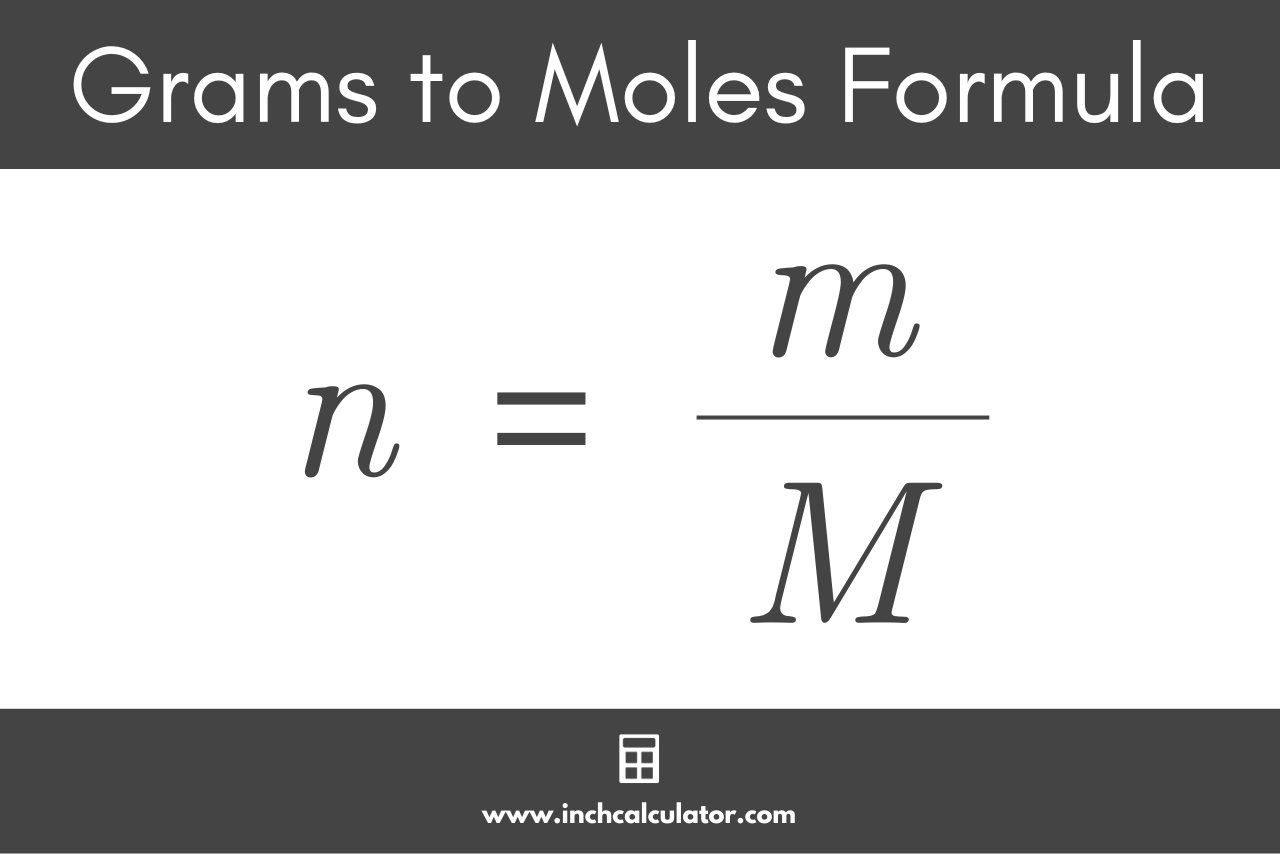# Grams to Moles Calculator

Convert grams to moles and moles to grams using our g to mol conversion calculator below.

grams
moles

## Quantity:

moles

### Molar Mass

g/mol
Learn how we calculated this below

## How to Convert Moles to Grams

Grams and moles are both units used in chemistry to measure matter in different ways. A gram is an SI unit of measurement for mass equal to 1/1,000 of a kilogram, and the mole is the SI base unit used to represent the quantity of a substance.

One mole is equal to 6.02214076 × 1023 elementary units of matter, such as atoms or molecules. Because that’s such a large number, it’s almost always represented using scientific notation.

Moles count the number of atoms or molecules in a sample, while grams represent the weight of the sample.

Moles are normally used in chemical formulas to represent the relative amount of each substance in the formula – in other words, how many moles of each reactant are needed to make a certain number of moles of product.

Grams are used to measure out the reactants and to check the yield of the products in a reaction.

At this point, you might be wondering how to convert moles, which are used in chemical formulas, to grams, which is how much of each compound you’ll need to carry out the reaction in a laboratory.

### Step One: Find the Molar Mass

The first step in the conversion is to find the molar mass of the compound. Because every substance is composed of different atomic elements, the atomic mass of each element in a compound or molecule is added together to determine its molar mass.

Molar mass, represented in g/mol, will be needed along with the formula below to convert moles to grams or vice versa.

You can either use the molar mass of the entire substance, or you can calculate the molar mass of the substance by adding together the atomic masses of each element that makes up that substance. You can use a molar mass calculator for this calculation if you don’t have a reference handy. However, the calculator above will automatically calculate the molar mass for you.

### Step Two: Use the Moles to Grams Formula

The formula used to convert moles to grams is:

m = n × M

Thus the mass m of substance in grams is equal to the quantity n in moles multiplied by the molar mass M of the substance in g/mol.For example, let’s calculate the mass of 2 moles of table salt, sodium chloride or NaCl. The molar mass of sodium chloride is 58.44 g/mol, which is the sum of the atomic masses of 1 mole of sodium (Na, 22.99 g/mol) and 1 mole of chlorine (Cl, 35.45 g/mol).

Two moles of table salt, NaCl, would be twice this amount:

m = 2 × 58.44
m = 116.88 g

So, the mass of 2 moles of sodium chloride is 116.88 grams.

You can also use our mole calculator to find the quantity of a substance.

## How to Convert Grams to Moles

You’ll need a grams to moles formula to find the moles of a substance you have. For example, once you have carried out a reaction, you will weigh your product to find out how many grams have been produced. You can then convert grams back to moles to check the yield of the reaction.

### Grams to Moles Formula

To convert the mass in grams back to the quantity of substance in moles, we simply need to reverse the formula above. The g to mol formula is:

n = m / M

Thus, the quantity of substance n in moles is equal to the mass m in grams divided by the molar mass M in g/mol.For example, let’s calculate the number of moles equal to 120 grams of aluminum oxide, Al2O3. Note that the molar mass of aluminum oxide is 101.96 g/mol.

n = 120 g / 101.96
n = 1.18 mol

So, in this example, 120 grams of aluminum oxide equals 1.18 moles.

Did you know you can also convert liters and atoms to moles as well?

## References

1. National Institute of Standards and Technology, SI Units - Mass, https://www.nist.gov/pml/owm/si-units-mass
2. International Bureau of Weights and Measures, SI base unit: mole (mol), https://www.bipm.org/en/si-base-units/mole
3. National Institute of Standards and Technology, NIST Guide to the SI, Chapter 8, https://www.nist.gov/pml/special-publication-811/nist-guide-si-chapter-8